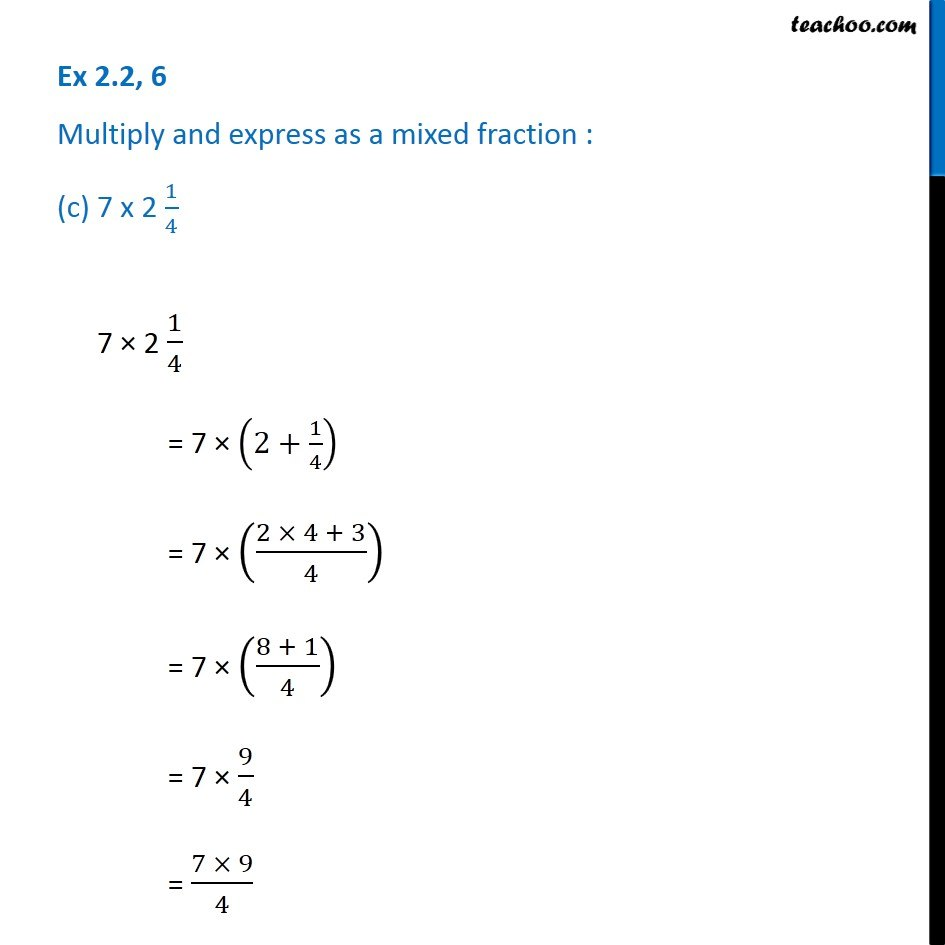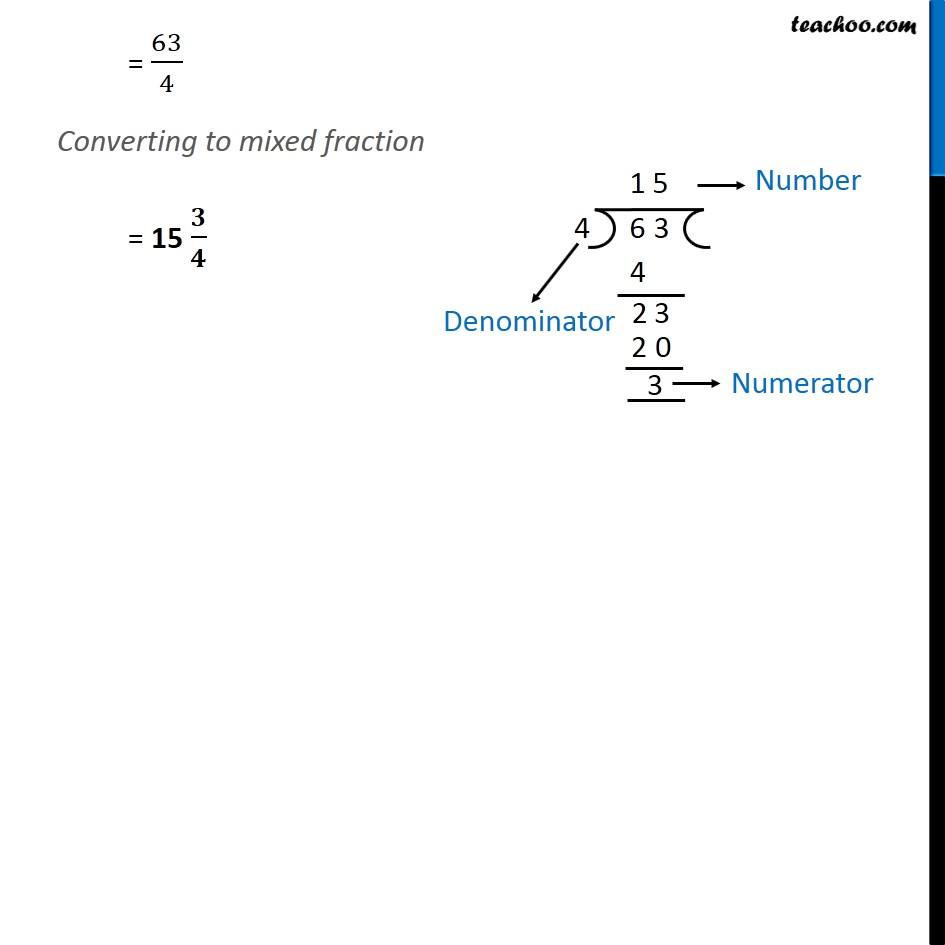1. Chapter 2 Class 7 Fractions and Decimals
2. Serial order wise
3. Ex 2.2

Transcript

Ex 2.2, 6 Multiply and express as a mixed fraction : (c) 7 x 2 1/4 7 × 2 1/4 = 7 × (2+1/4) = 7 × ((2 × 4 + 3)/4) = 7 × ((8 + 1)/4) = 7 × 9/4 = (7 × 9)/4 = 63/4 Converting to mixed fraction = 15 𝟑/𝟒